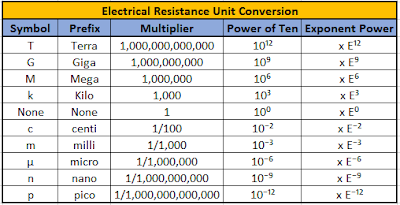## Ohm Converter

Ohm converter or Resistance unit converter will help you to convert resistance units in easy way. Also, the below article gives details about the need of Ohm converter.

### Ohm Converter or Resistance Unit Converter

An ohm converter or resistance unit converter has been provided. This tool will help you to perform easily and quickly conversion of different resistance measurement units to another resistance measurement unit with accuracy.

Please click on below symbol and download the “Ohm converter or Resistance Unit Converter” in excel format and use in your computer, laptop, tablet or mobile.Ohm Converter

### Need of Ohm Converter

Let define the electrical resistance, “the opposition or obstruction to the path of an electric current through a component is called as electrical resistance”.

Ohm is the SI unit of electrical resistance and it is denoted by “Ω”.

The V, A and Ω are the standard units of electrical measurement for Voltage, Current and Resistance respectively.

On the basis of international metric system (SI system) these electrical units of measurement is decided. Also, from SI base units other generally used electrical units being taken out.

Electrical Resistance is the resistance between two points of a conducting material, produces a current of 1 A, when a constant potential difference of 1 V is applied.

After performing calculations to measure the resistance value, we often want to convert the measured resistance unit to some other measured resistance unit, so we can carry out the calculation process in easy way.

Most of time because of human error while converting form one unit to other unit we get wrong results. This wrong results will further affect our calculations.

Many time while performing calculations for designing the circuit, and the measured quantities or results are very large or very small, then we have to use multiples or sub-multiples of these standard electrical measuring units.

Many times we get resistance results such that, it can be lower than 0.01Ω or higher than 1,000,000Ω. By using standard unit’s multiples and submultiples we can avoid to write many zeros for defining the place of the decimal point.

Below tables gives Electrical Resistance Unit Conversion for better understanding the conversion process and same conversion technique has been used by the Electrical Resistance Converter.Ohm Converter

### Conclusion

The conversion of units is a mostly used step in performing calculations. Many times Electrical resistance unit is needed to be convert from one form to another form i.e. unit, to perform the calculation process in easy steps. The tool presented here, “Ohm Converter or Resistance Unit Converter”, will help you to get accurate and fast result.